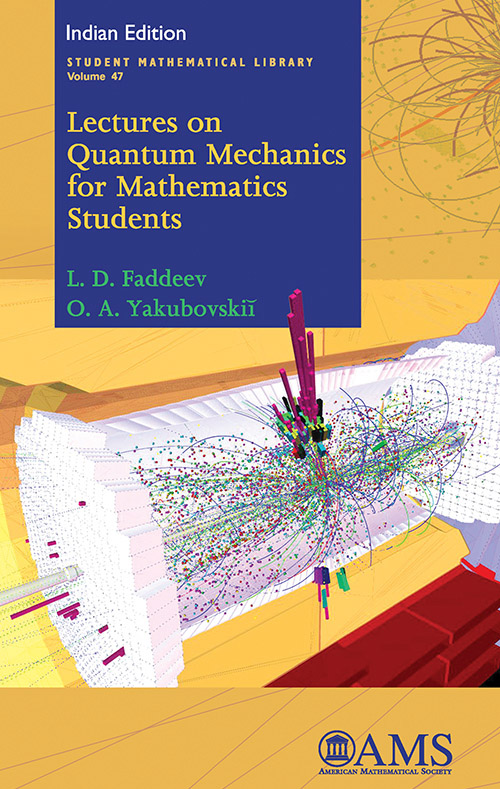## Lectures on Quantum Mechanics for Mathematics Students

#### Price: 1525.00

This book is based on notes from the course developed and taught for more than 30 years at the Department of Mathematics of Leningrad University. The goal of the course was to present the basics of quantum mechanics and its mathematical content to students in mathematics. This book differs from the majority of other textbooks on the subject in that much more attention is paid to general principles of quantum mechanics. In particular, the authors describe in detail the relation between classical and quantum mechanics. While selecting particular topics, the authors emphasize those that are related to interesting mathematical theories. In particular, the book contains a discussion of problems related to group representation theory and to scattering theory.

This book is rather elementary and concise, and it does not require prerequisites beyond the standard undergraduate mathematical curriculum. It is aimed at giving a mathematically oriented student the opportunity to grasp the main points of quantum theory in a mathematical framework.

L. D. Faddeev: Steklov Mathematical Institute, St. Petersburg, Russia,
O. A. Yakubovskii: St. Petersburg University, St. Petersburg, Russia

• Preface 12
• Preface to the English edition 14
• The algebra of observables in classical mechanics 16
• States 21
• Liouville’s theorem, and two pictures of motion in classical mechanics 28
• Physical bases of quantum mechanics 30
• A finite-dimensional model of quantum mechanics 42
• States in quantum mechanics 47
• Heisenberg uncertainty relations 51
• Physical meaning of the eigenvalues and eigenvectors of observables 54
• Two pictures of motion in quantum mechanics. The Schrödinger equation. Stationary states 59
• Quantum mechanics of real systems. The Heisenberg commutation relations 64
• Coordinate and momentum representations 69
• “Eigenfunctions” of the operators Q and P 75
• The energy, the angular momentum, and other examples of observables 78
• The interconnection between quantum and classical mechanics. Passage to the limit from quantum mechanics to classical mechanics 84
• One-dimensional problems of quantum mechanics. A free one-dimensional particle 92
• The harmonic oscillator 98
• The problem of the oscillator in the coordinate representation 102
• Representation of the states of a one-dimensional particle in the sequence space l2105
• Representation of the states for a one-dimensional particle in the space D of entire analytic functions 109
• The general case of one-dimensional motion 110
• Three-dimensional problems in quantum mechanics. A three-dimensional free particle 118
• A three-dimensional particle in a potential field 119
• Angular momentum 121
• The rotation group 123
• Representations of the rotation group 126
• Spherically symmetric operators 129
• Representation of rotations by 2×2 unitary matrices 132
• Representation of the rotation group on a space of entire analytic functions of two complex variables 135
• Uniqueness of the representations Dj 138
• Representations of the rotation group on the space L2(S2) Spherical functions 142
• The radial Schrödinger equation 145
• The hydrogen atom. The alkali metal atoms 151
• Perturbation theory 162
• The variational principle 169
• Scattering theory. Physical formulation of the problem 172
• Scattering of a one-dimensional particle by a potential barrier 174
• Physical meaning of the solutions ? 1 and ? 2  179
• Scattering by a rectangular barrier 182
• Scattering by a potential center 184
• Motion of wave packets in a central force field 190
• The integral equation of scattering theory 196
• Derivation of a formula for the cross-section 198
• Abstract scattering theory 203
• Properties of commuting operators 212
• Representation of the state space with respect to a complete set of observables 216
• Spin 218
• Spin of a system of two electrons 223
• Systems of many particles. The identity principle 227
• Symmetry of the coordinate wave functions of a system of two electrons. The helium atom 230
• Multi-electron atoms. One-electron approximation 232
• The self-consistent field equations 238
• Mendeleev’s periodic system of the elements 241
• Lagrangian formulation of classical mechanics 246

`